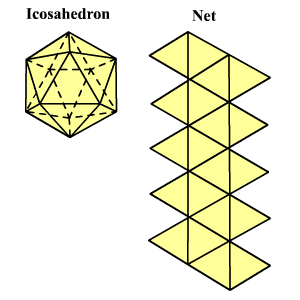# Platonic Solids

The platonic solids (or regular polyhedra) are convex with faces composed of congruent , convex regular polygons .  The mathematician Euclid proved that there are exactly five such solids.  They are the tetrahedron, cube, octahedron, dodecahedron and icosahedron.

The tetrahedron has $6$ faces.  Each is an equilateral triangle .  It also has $6$ edges and $6$ vertices.  At each vertex three edges meet.

Surface Area $=\sqrt{3}{e}^{2}$

Volume $=\frac{\sqrt{2}}{12}{e}^{3}$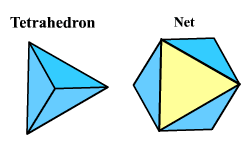The cube has $6$ faces.  Each is a square .  It also has $12$ edges and $8$ vertices.  At each vertex three edges meet.

Surface Area $=6{e}^{2}$
Volume $={e}^{3}$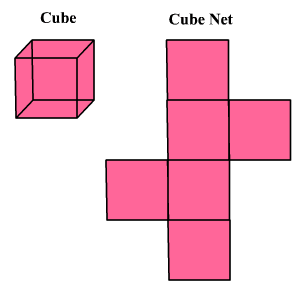The octahedron has $8$ faces.  Each is an equilateral triangle.  It also has $12$ edges and $6$ vertices.  At each vertex four edges meet.

Surface Area $=2\sqrt{3}{e}^{2}$

Volume $=\frac{\sqrt{2}}{12}{e}^{3}$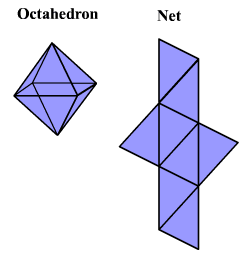The dodecahedron has $12$ faces.  Each is a regular pentagon.  It also has $30$ edges and $20$ vertices.  At each vertex three edges meet.

Surface Area $=3\sqrt{25+10\sqrt{5}}{e}^{2}$

Volume $=\frac{15+7\sqrt{5}}{4}{e}^{3}$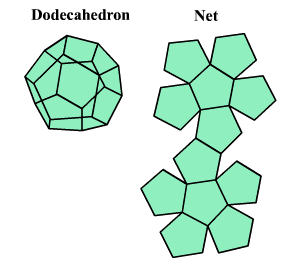The icosahedron has $20$ faces.  Each is an equilateral triangle.  It also has $30$ edges and $12$ vertices.  At each vertex five edges meet.

Surface Area $=5\sqrt{3}{e}^{2}$

Volume $=\frac{5}{12}\left(3+\sqrt{5}\right){e}^{3}$